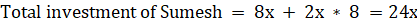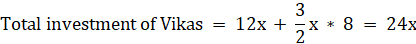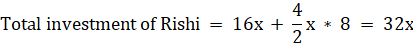Question of The Day01-08-2022

Three friends Sumesh, Vikas, and Rishi started a business with their monthly investment in the ratio of 2 : 3 : 4. After 4 months, Sumesh invested the same amount as before, and Vikas and Rishi, did only half of their investment. Find the ratio of their profit at the end of year?

Correct Answer : b ) 3 : 3 : 4

Explanation :

The ratio of investment of Sumesh, Vikas and Rishi = 2 : 3 : 4

Let us take the initial monthly investment be 2x, 3x, and 4x

After 4 months investment will be

Investment of Sumesh = 4 * 2x = 8x

Investment of Vikas = 4 * 3x = 12x

Investment of Rishi = 4 * 4x = 16x

After 4 months Sumesh invests the same amount as before and Vikas and Rishi withdrew half of their investment.

Total investment after 12 months,Ratio of profit sharing at the end of year = Ratio of total investment

= 24x : 24x : 32x = 3 : 3 : 4

Hence, (b) is the correct answer.

This question is based on the concept of partnership and the ratio and proportion.0